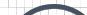Informative Information for the Uninformed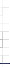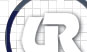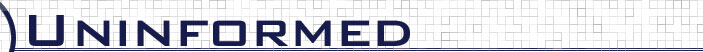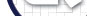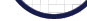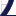Vol 5» 2006.Sep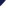# BayesCompare Metric

BayesCompare was created as an attempt to use a well understood rule to classify 802.11 implementations. In document classification, the problem is that of given a set W of words appearing in a document, classify the document as belonging to one of several categories. One takes the category to be the category C that maximizes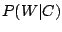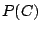. The conditional probabilitycomes from a training set of documents known to be in category C. If we take W to be the set of durations occurring in a given packet capture that we want to identify by implementation thenbecomes the probability of W occurring in a capture given that the capture comes from 802.11 implementation C.

Classification in this manner is only as good as the training set (print database). A given training set may not yet know that implementation C can produce duration D. Hence, which is approximated from the training set, is zero when W contains D even though W may contain another duration that uniquely identifies C. Further, approximatingis problematic, as it is the probability of seeing a given 802.11 implementation. One might approximate it by perhaps chipset market share but this would be somewhat inaccurate because it ignores the fact that a device driver is part of an 802.11 implementation we wish to identify. Getting an accurate approximation of it is difficult so we chose to ignore it. This of course puts the metric at a slight disadvantage compared to the other metrics, as we shall see.

Let X be an 802.11 implementation for which a fingerprint exists in the print database. Let L be the duration fingerprint arising from an input pcap file. We want the probability that the input pcap file originated with implementation X given L: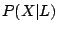. Using Bayes rule,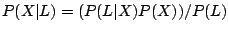. The idea here is to use these conditional probabilities to rank the degree of a match between L and each fingerprint in the print database. Therefore, we did not compute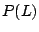for a given input pcap as it is constant across all fingerprints in the database. Of course probability P(X) is not constant across all fingerprints but computing it is problematic, as discussed above. Therefore, we didn't compute it as part of the conditional probability. Further, to simplify things, we approximated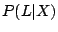as the product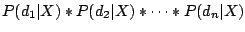where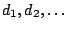are the distinct durations that appear in L. This assumes that the individual duration values in L occur independently which one can argue isn't true since the durations occur in sequence for certain control frames, for instance, duration values in ACK, RTS and CTS frames. But as mentioned previously, control frames are ignored in fingerprints.

If L denotes the fingerprint arising from an input pcap file and R a fingerprint in the print database then we take the preceding product to be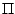R.duration_ratio(d) where d ranges over all durations in L. And when taking into account packet types in which durations occur, it becomesR.duration_ratio(p, d) where p and d range over all packet types and durations respectively where duration d occurs in a packet of type p in L.

Figure 4.7: BayesCompare duration value only analysis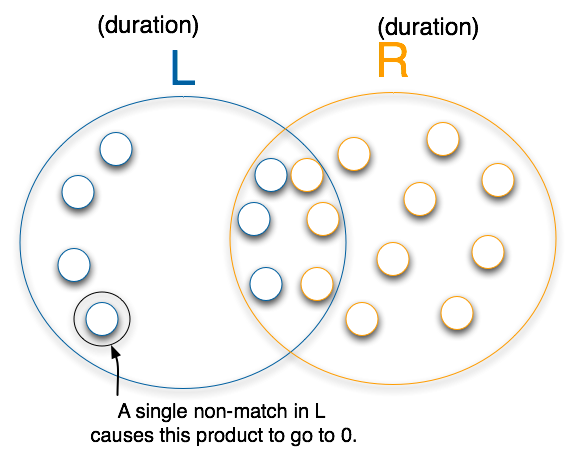ret = 1.0
for every duration-value d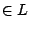ret *= R.duration_ratio(d)
return ret;



Figure 4.8: BayesCompare (packet_type, duration) analysis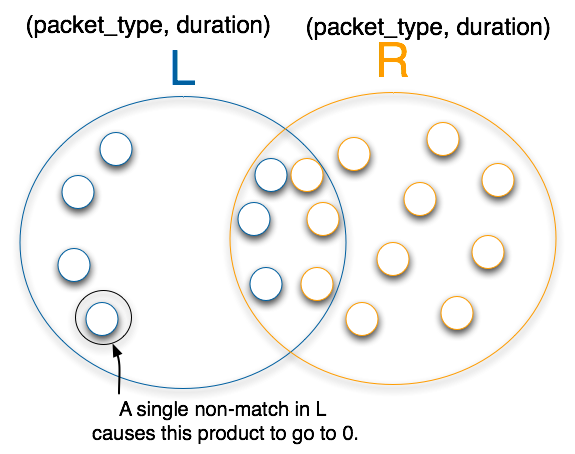ret = 1.0
for every packet_type p, duration-value dret *= R.duration_ratio(p, d)
return ret;